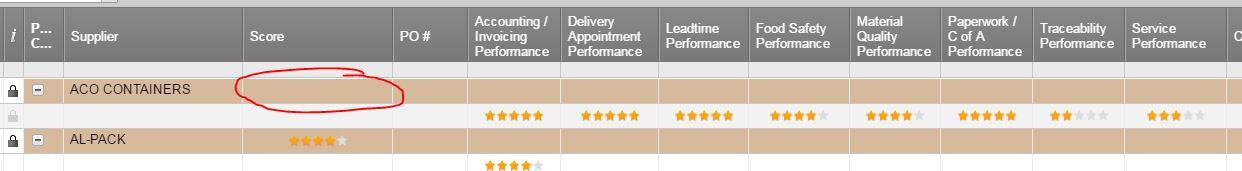#### Welcome to the Smartsheet Forum Archives

The posts in this forum are no longer monitored for accuracy and their content may no longer be current. If there's a discussion here that interests you and you'd like to find (or create) a more current version, please Visit the Current Forums.

# Using formula to average columns with rating stars

Hi Folks,

See my screen shot below.

I am trying to get score cell circled in red below to look at the star rating symbols in the child rows and provide the average of the child rows in the score cell.

I am assuming that 0 stars equals 0%, 1 star equals 20% etc..........

Help pointing me in the correct direction would be great.

Thanks

Jon• Jon,

The Symbol columns match to a friendly name. You can determine those values for formulas by selecting the drop-down menu and looking at the values.

In your case: "Empty", "One", "Two", "Three""Four""Five"

I recommend consulting the Formulas Help Article found here:

• Expanding on this, create another column to be hidden later called "Numeric Score" to the right of your Score Column. Put in a formula to translate the Score values to a number, like this:

=IF(Score2 = "Empty", 0, IF(Score2 = "One", 1, IF(Score2 = "Two", 2, IF(Score2 = "Three", 3, IF(Score2 = "Four", 4, IF(Score2 = "Five", 5, 0))))))

Next, for the summary row of this "Numeric Score" column, get the average and round it to a whole number:

=ROUND(AVG(CHILDREN()))

In the summary row for the Score column that you circled in red, write a formula to take this averaged number and turn it back into the Star Text:

=IF([Numeric Score]1 = 0, "Empty", IF([Numeric Score]1 = 1, "One", IF([Numeric Score]1 = 2, "Two", IF([Numeric Score]1 = 3, "Three", IF([Numeric Score]1 = 4, "Four", IF([Numeric Score]1 = 5, "Five"))))))

Optionally right click on the "Numeric Score" column and choose hide.

• It looks from your example that you are going to have to make a summary column that would tally each of your columns... as you have several columns with stars. You would have to convert each of your stars text counts of One, Two, etc. to numbers 1, 2 and then sum them and average them to get your score for the overall star box and then convert that to the text necessary for the star rating.

This discussion has been closed.